## iPhone Screenshots

•••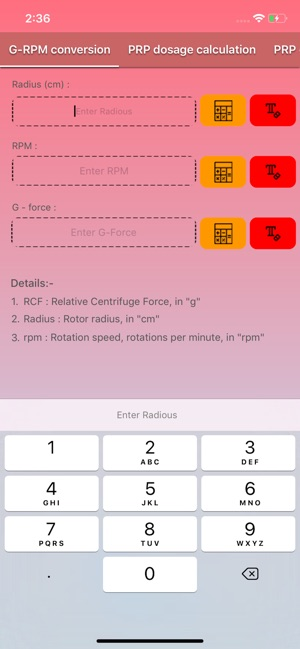•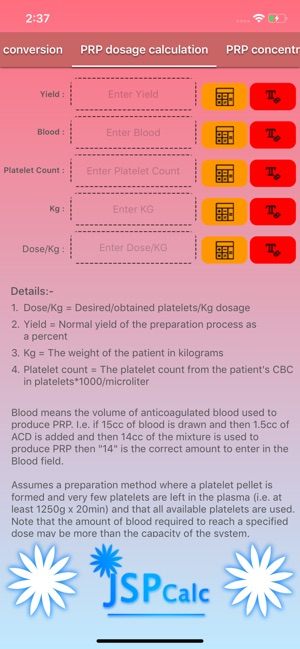•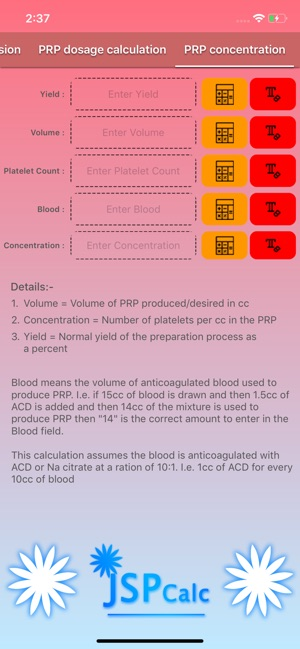•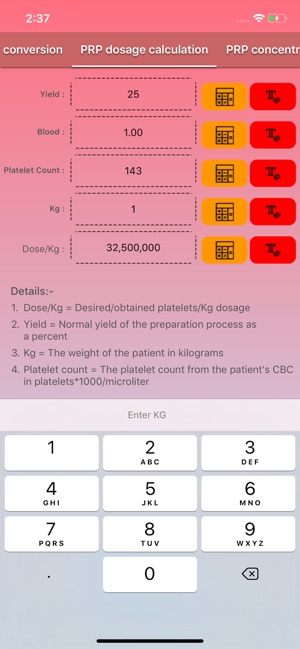•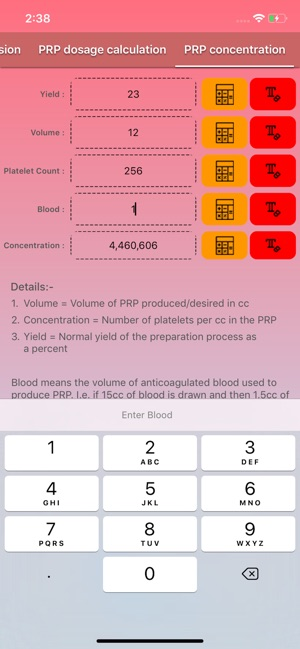## Description

The first calculator converts RPM (revolutions per minute) to RCF (relative centrifugal force, g-force). This is necessary when the user knows the g-force required for a prep, but their centrifuge is calibrated in RPM. The calculator can be used to determine any of the three variables from the other two.

r [mm] is the Rotational Radius in millimeters (Rotational Diameter could also be used instead of Rotational Radius!).

The PRP dosage calculator allows a user to calculate the dose or amount of blood required for a dose of PRP treatment. It assumes blood is anticoagulated with ACD at a 1:10 ratio and that the user knows the yield of their PRP preparation process.

This app calculates the Relative Centrifugal Force (RCF) for given Rotational Radius (r [mm]) and Rotational Speed (N [rpm]).

Calculated Relative Centrifugal Force (RCF) is measured in times of "g", a dimensionless quantity.

N [rpm] is the Rotational Speed in revolutions per minute.

This app also calculates the Rotational Speed (N [rpm]) for given Rotational Radius (r [mm]) (or Rotational Diameter) and Relative Centrifugal Force (RCF).

## Information

Seller
Joyce Gross
Size
1.8 MB
Category
Productivity
Compatibility

Requires iOS 11.0 or later. Compatible with iPhone, iPad, and iPod touch.

Languages

English

Age Rating
Rated 4+
Copyright
© Atmiya Info
Price
Free

## Supports

•